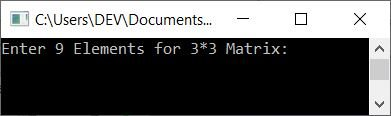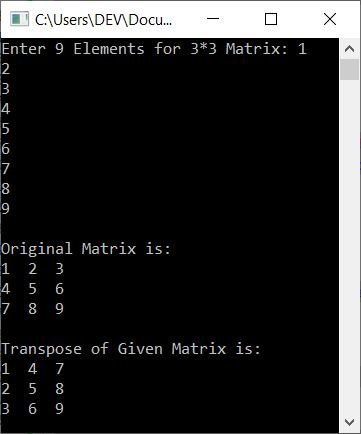# C++ Program to Transpose a Matrix

In this article, you will learn and get code to find the transposition of a matrix entered by a user using a C++ program. Here is the list of programs available in this article:

### How to Find the Transpose of a Matrix

The transposition of a matrix can be calculated by interchanging rows and columns. For example, if the value of a matrix, say Matrix A, is:

```1  2  3
4  5  6
7  8  9```

Then its transpose (AT) will be:

```1  4  7
2  5  8
3  6  9```

## In C++, find the transpose of a 3*3 matrix

To transpose any matrix in C++ programming, you have to ask the user to enter elements of the matrix. To transpose that matrix, simply replace row with column and column with row. Then display or print the transposition of the given matrix on the output, as shown here in the following program:

```#include<iostream>
using namespace std;
int main()
{
int mat, i, j, matT;
cout<<"Enter 9 Elements for 3*3 Matrix: ";
for(i=0; i<3; i++)
{
for(j=0; j<3; j++)
cin>>mat[i][j];
}
cout<<"\nOriginal Matrix is:\n";
for(i=0; i<3; i++)
{
for(j=0; j<3; j++)
cout<<mat[i][j]<<"  ";
cout<<endl;
}
// copying the transpose of given matrix to matT[][]
for(i=0; i<3; i++)
{
for(j=0; j<3; j++)
matT[j][i] = mat[i][j];
}
cout<<"\nTranspose of Given Matrix is:\n";
for(i=0; i<3; i++)
{
for(j=0; j<3; j++)
cout<<matT[i][j]<<"  ";
cout<<endl;
}
cout<<endl;
return 0;
}```

This program was built and runs under the Code::Blocks IDE. Here is its sample run:Now supply the input as nine elements. Here is the sample output after supplying 9 elements:The following block of code:

```for(i=0; i<3; i++)
{
for(j=0; j<3; j++)
matT[j][i] = mat[i][j];
}```

copies the transpose of the given matrix mat[][] to matT[][]. Therefore the dry run of this block of code with the user inputs 1, 2, 3, 4, 5, 6, 7, 8, and 9 goes like this:

• Because the user enters these 9 elements as inputs, they get stored in mat[][] in this way:
• mat=1
• mat=2
• mat=3
• mat=4
• mat=5
• and so on up until
• mat=9
• Let's get to the code that copies the matrix's transpose to matT[][]. Initially, 0 gets initialized to i, and the condition i<3 or 0<3 evaluates to be true. Therefore, program flow goes inside the loop.
• Inside the loop, there is another for loop. So 0 gets initialized to j, and the condition j<3 or 0<3 evaluates to be true, therefore program flow goes inside this loop, and mat[i][j] or mat or 1 gets initialized to matT[j][i] or matT. So matT=1
• Now the program flow goes to the update part of the inner for loop and increments the value of j. As a result, j=1 and the condition j<3 or 1<3 evaluates to true once more.
• Therefore, program flow goes inside the loop again, and mat[i][j] or mat or 2 gets initialized to matT[j][i] or matT. So mat=2
• Now the program flow again goes to the update of the inner for loop and increments the value of j. So j=2 and the condition j<3 evaluates to be true again, therefore program flow goes inside the loop again. This process continues until the condition is evaluated as false.
• When the condition of the inner for loop evaluates to be false, then program flow goes to the update part of the outer for loop and increments the value of i. So i=1, and the condition i<3 or 1<3 evaluates to be true again, therefore program flow goes inside the loop. This process continues until the condition is evaluated as false.
• The transpose of matrix mat[][] is thus copied to matT[][].
• As a result, simply print the value of matT[][].

### Allow the user to specify the dimensions of the matrix

This program is similar to the previous program. The only difference is that here we've allowed the user to enter the dimension of the matrix along with its elements before finding and printing the transposition of the given matrix:

```#include<iostream>
using namespace std;
int main()
{
int mat, matT;
int matRow, matCol, i, j;
cout<<"Enter Row and Column Size of Matrix: ";
cin>>matRow>>matCol;
cout<<"Enter "<<matRow*matCol<<" Elements for "<<matRow<<"*"<<matCol<<" Matrix: ";
for(i=0; i<matRow; i++)
{
for(j=0; j<matCol; j++)
cin>>mat[i][j];
}
cout<<"\nOriginal Matrix is:\n";
for(i=0; i<matRow; i++)
{
for(j=0; j<matCol; j++)
cout<<mat[i][j]<<"  ";
cout<<endl;
}
// copying the transpose of given matrix to matT[][]
for(i=0; i<matRow; i++)
{
for(j=0; j<matCol; j++)
matT[j][i] = mat[i][j];
}
cout<<"\nTranspose of Given Matrix is:\n";
for(i=0; i<matCol; i++)
{
for(j=0; j<matRow; j++)
cout<<matT[i][j]<<"  ";
cout<<endl;
}
cout<<endl;
return 0;
}```

Here is its sample run with user inputs 2 and 4 as dimensions. That is, 2 as row size and 4 as column size, then  1, 2, 3, 4, 5, 6, 7, 8 as its 8 elements. Here is the sample run with these inputs:#### The same program in different languages

C++ Quiz

« Previous Program Next Program »